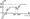# Control Systems July/August 2005

Note: Answer any FIVE full questions.

1.  (a) For what purpose feedback is used in control systems? .Mention the effects of feedback on i) stability ii) overall gain iii) disturbance and iv) sensitivity of control systems.

(b) Draw the F-V analogous mechanical system for the electrical circuit shown in fig.l, writing the loop equations for the electrical circuit, then transforming them to their mechanical analog.2.  (a) Illustrate how to perform the following in connection with block diagram reduction techniques:

i)     moving a summing point ahead of a block and behind a block

ii) moving a take off point ahead of a block and behind a block

iii)   Transforming a non unity feedback to a unity feedback.

(b) Explain Masons gain formula. Use it to determine the transmittance of the flow graph shown in Fig. 2.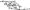3.  (a) For the two port network shown in Fig.3, obtain the transfer functions

i) and ii)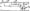(b) For a unity feedback control system with G{s) write the output response to a

unit step input. Determine:

i)    The response at t = 0.1 sec

ii)   Maximum value of the response and the time at which it occurs

iii)   Setting time.

4. (a) Obtain expressions for i) peak resonance ii) resonance frequency and iii) band width of a proto type second order system.

(b) For the flow graph shown in fig.4, mention the type number and order of the system and determine the steady state errors for step and ramp inputs e(t) — r(t)  y(t).5.  (a) The polynomial P(S) — 54 + 253 + 352 + 5 + l has all its roots in LHS of a plane. Use R-H criterion to determine the number of roots of P(S) lying between S-\ and S = -1

(b) The block diagram of a feedback control system is shown in fig.5. Apply RH criterion to determine the range of K for stability if G(S) — -t;—&■„ — – (lfl Marks)6.  (a) For a unity feed back system G(S) -determine the range of K for the system to be stable using Nyquist criterion.

(b) For the polar plot shown in fig. 6

i)    determine the gain margins in dB and the phase margins if OA — -0.5, OB = -1, OC = -2, OD = -2.5 OE – -0.866+J0.5 and OF = -0.643-J0.766.

ii)   Complete the Nyquist, plot and determine whether the system is stable, if all poles are in LH of s plane.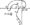7. (a) As applied to root locus, explain how to

i)     determine angle of departure or arrival from a complex pole or zero

ii)   determine the breakaway or breakin points if they are present

iii)   calculate K on a given point on the root locus.                          (10 Marks)

(b) The root locus for G(S)H(S) is shown in Fig. 7.

i)    What value of K gives a damping ratio 6 = 0.707 for the two poles nearest the JW axis when die system is operated closed loop?

ii)   What is the setting time for the closed loop system with the two poles adjusted to have S = 0.707?

iii)   What K makes the closed loop system go unstable?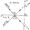8.  (a) Draw bode plot for the system shown in fig.8. Determine gain margin and phase margin.

(b) Estimate the transfer function from the bode plot shown in fig.9. Digits on line indicate slope in dB.